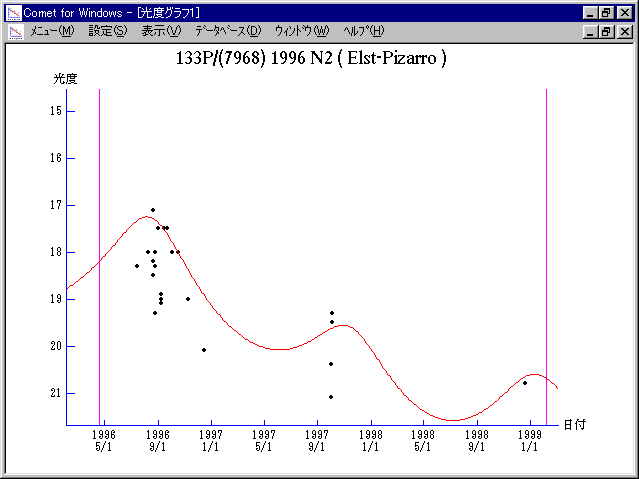# \$B%(%k%9%H(B-\$B%T%5%mWB@1(B

133P/(7968) Elst-Pizarro (1996)###\$B%W%m%U%#!<%k(B

 \$BId9f(B 133P/(7968) 1996 N2 \$BH/8+F|(B 1996\$BG/(B7\$B7n(B14\$BF|(B \$BH/8+8wEY(B 18.3\$BEy(B \$BH/8+ Eric W. Elst, Guido Pizarro (European Southern Observatory)

###\$B50F;MWAG(B

```   The following improved orbital elements by Kenji Muraoka, are
from 152 observations 1985 to 1996, perturbations by 9 Plantes,
Moon and 5 minor planets were taken into account.
The mean residual is +/- 0.61 arc seconds.

Epoch  =  1996 Apr. 27.0  TT       JDT = 2450200.5
T  =  1996 Apr. 18.38774       +/- 0.02777 (m.e.) TT
Peri. =  133.30399                +/- 0.00721
Node  =  160.26603                +/- 0.00086   (2000.0)
Incl. =    1.38424                +/- 0.00009
q  =    2.6275500              +/- 0.0000313 AU
e  =    0.1674936              +/- 0.0000100
a  =    3.1561918              +/- 0.0000170 AU
n  =    0.17577576             +/- 0.00000142
P  =    5.607                  +/- 0.0000452  years
```

###\$B@1?^(B1995 Jan. 3 - 1996 Dec. 231996 Feb. 7 - 1997 Dec. 18

###\$B8wEYJQ2=(B

```        m1 = 9.1 + 5 log\$B&\$(B + 16.5 log r
```##### \$B50F;MWAG\$OB<2,7r<#;a\$N7W;;\$K\$h\$k\$b\$N\$G\$9!#(B \$B@1?^\$O(B StellaNavigator Ver.2.0 for Windows (\$B%"%9%H%m%"!<%D(B \$BJTCx(B / \$B%"%9%-!<=PHG6I4)(B) \$B\$G:n@.\$7\$?\$b\$N\$G\$9!#(B \$B8wEY%0%i%U\$O(BComet for Windows\$B\$G:n@.\$7\$?\$b\$N\$G\$9!#(B